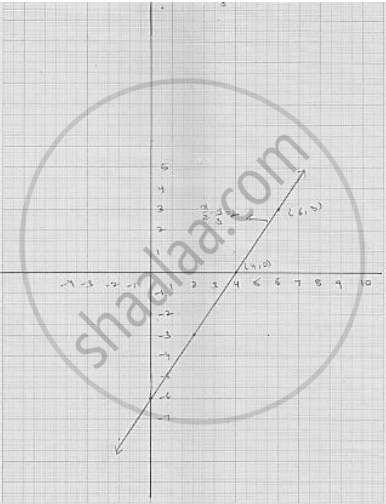# Draw the Graph of the Following Linear Equation in Two Variable :  X / 2 - Y/ 3 = 2 - Mathematics

Draw the graph of the following linear equation in two variable :  x / 2 - y/ 3 = 2

#### Solution

We have

 x / 2 - y/ 3 = 2

⇒  (3x - 2y) /6 = 2

⇒  3x - 2y - 12

⇒ 3x = 12 + 2y

⇒ x + (12 + 2y )/3

putting   y = - 6 , we get   x = (12 + 2 (-6 ))/3 = 0

Putting  y = - 3 , we get   x  = (12 + 2 (-3))/3  = 2

Putting  y  = 0 we get  x  = (12 + 0 )/3  = 4

Thus, we get the following table giving the two points on the line represented by the
equation   x / 2 - y/ 3 = 2

Graph for the equation    x / 2 - y/ 3 = 2Concept: Graph of a Linear Equation in Two Variables
Is there an error in this question or solution?

#### APPEARS IN

RD Sharma Mathematics for Class 9
Chapter 7 Linear Equations in Two Variables
Exercise 7.3 | Q 1.6

Share